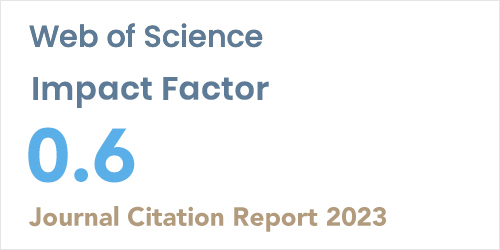ISSN: 1304-7191 | E-ISSN: 1304-7205
Volume: 41 Issue: 4 Year: 2023A galerkin-type method for solutions of pantograph-type volterra-fredholm integro-differential equations with functional upper limit
1Akdeniz University, Department of Mathematics, ANTALYA
Sigma J Eng Nat Sci 2020; 38(2): 995-10005

#### Abstract

In this study, we present a Galerkin-type method for obtaining approximate solutions of linear Volterra-Fredholm delay integro-differential equations with a functional upper limit under mixed conditions. The method gives an approximate solution of the problem in power series form truncated after a certain term. Using an integer value N as the truncation point and making use of the matrix representations of a polynomial and its derivatives, we obtain the matrix form of the problem expressed in terms of the approximate solution polynomial. By applying inner product to these relations with monomials up to degree N and incorporating the mixed conditions, the problem is reduced to a system of linear algebraic equations. The approximate solution of the problem is then determined from this linear system. In addition, we discuss a way of improving an obtained approximate solution by means of its estimated error function. The presented scheme has the advantages of (1) being applicable to a wide range of problems including pantograph-type equations with or without Fredholm and Volterra integral terms, and (2) giving accurate results as demonstrated by applications to example problems taken from existing studies.